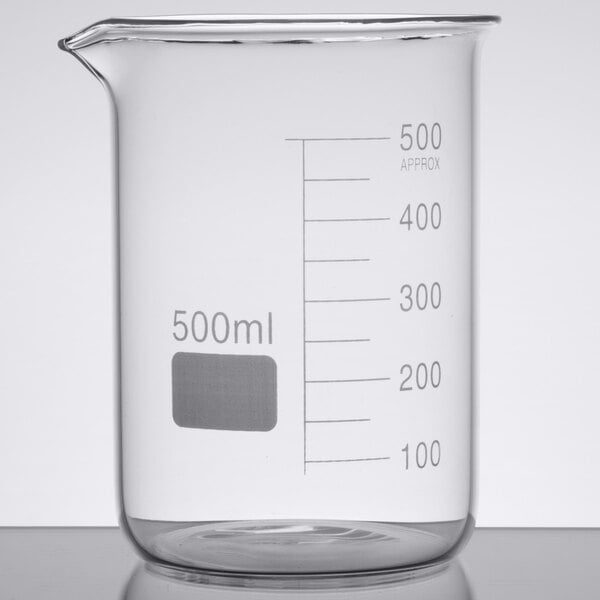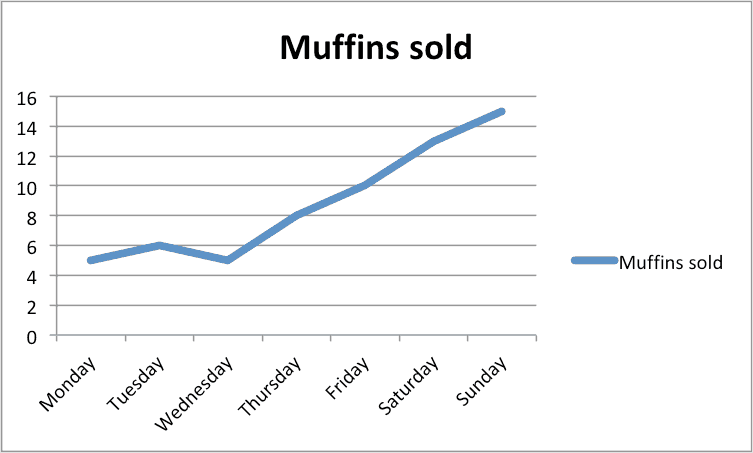Variables
Graphing
Scientific Method
Measurements
Tools
100

Anything that changes in an experiment.

What is a VARIABLE?

100

This belongs on the top of every graph you make.

What is a TITLE?

100

This step answers the original question.

What is a conclusion?

100

This is the basic unit for length in the metric system.

What is the meter?

100

The tool used to measure the length of an object.

What is a metric RULER or METER STICK?

200

The variable that you make different in order to test it.

What is an INDEPENDENT VARIABLE?

200

What is the name of the horizontal (side to side) axis?

What is X-AXIS?

200

An educated guess or prediction for what might happen in an experiment.

What is a HYPOTHESIS?

200

This is the basic unit for volume.

What is the liter?

200

The tool best used to measure the volume of a liquid.

300

The variable that you observe or measure during an experiment.

What is a DEPENDENT VARIABLE?

300

True or False: You don't need to label the bottom and side of a graph.

What is False?

300

Data given that is expressed in numbers

What is QUANTITATIVE DATA?

300

This data is gathered and described using one of our five senses

What is QUALITATIVE DATA?

300

A tool best used to measure the mass of an object.

What is a TRIPLE BEAM BALANCE?

400

Something that stays the same to keep an experiment fair.

What is a CONSTANT?

400

What is the name of the vertical (up and down) axis?

What is the Y-AXIS?

400

Numbers, observations, or information you collect in an experiment.

What is DATA?

400

A measure of how much 3D space an object takes up.

What is volume?

400What is this object called?

What is a BEAKER?

500

The number of variables that you can change in an experiment.

What is one?

500This is a picture of what type of graph?

What is a LINE GRAPH?

500

A list of things you do to perform the experiment(not Scientific Method)

What are PROCEDURES?

500

The amount of matter in an object.

What is mass?

500

Object used to look at objects to small for the naked eye to see.

What is a MICROSCOPE?

Click to zoom